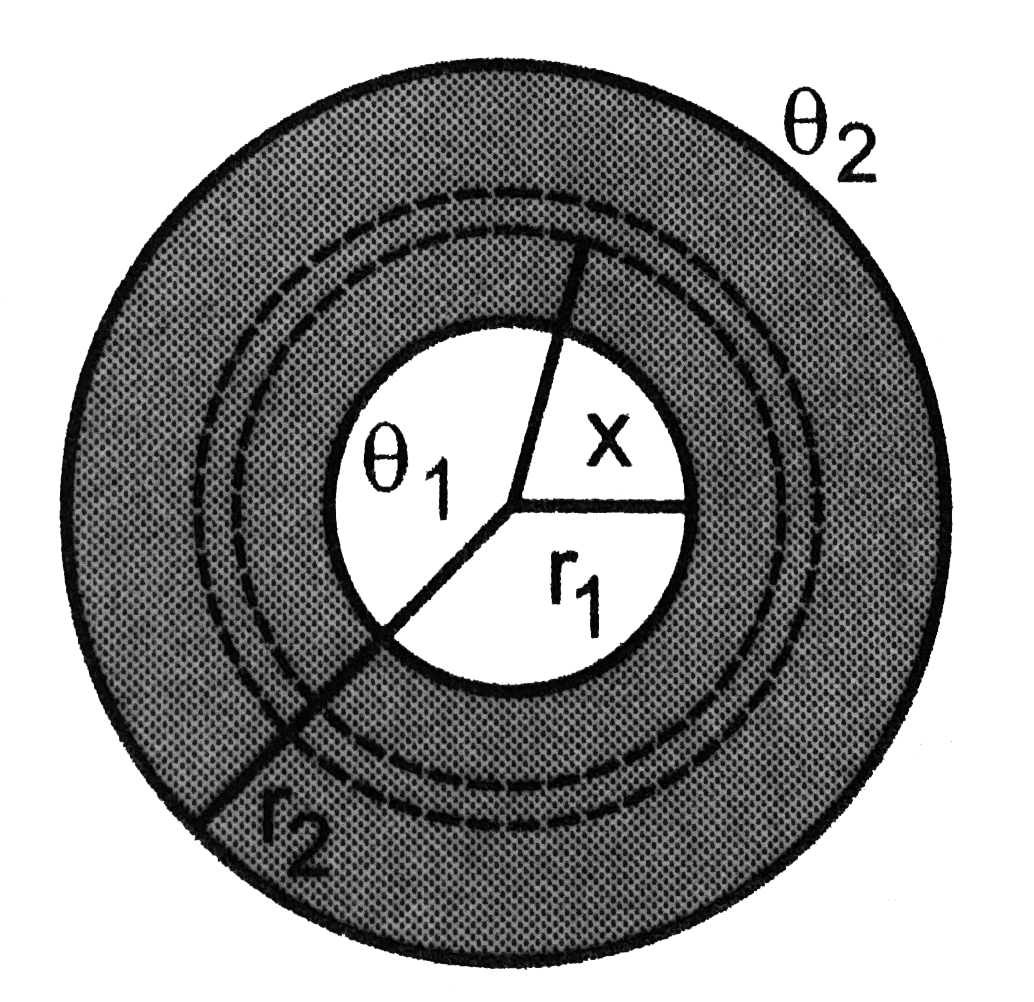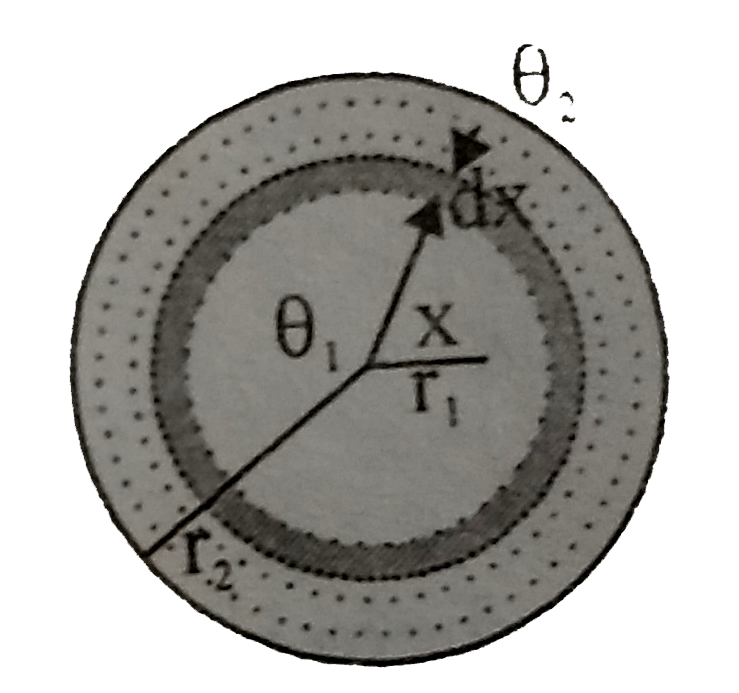# Two thin metallic spherical shells of radii r_(1) and r_(2) (r_(1)lt r_(2)) are placed with their centres coinciding. A material of thermal cond

23 views
in Physics
closed
Two thin metallic spherical shells of radii r_(1) and r_(2) (r_(1)lt r_(2)) are placed with their centres coinciding. A material of thermal conductivity K is filled in the space between the shells. The inner shells is maintained at temperature theta_(1) and the outer shell at temperature theta_(2) (theta_(1)lttheta_(2)). Calculate the rate at which heat flows radially through the material.by (90.1k points)

Let us draw two spherical shells of radii x and x+dx concentric with the given system. Let the temperature at these shells be theta and theta+d theta respectively. The amount of heat flowing radially inward through the material between x and x+dx is
(DeltaQ)/(Deltat)=(K4pix^(2)d theta)/(dx)
Thus, K4piint_(theta_(1))^(theta_(2))d theta=(DeltaQ)/(Deltat)int_(r_(1))^(r_(2))(dx)/(x^(2))
K4pi(theta_(2)-theta_(1))=(DeltaQ)/(Deltat)((1)/(r_(1))-(1)/(r_(2)))
(DeltaQ)/(Deltat)=(4piKr_(1)r_(2)(theta_(2)-theta_(1)))/(r_(2)-r_(1))